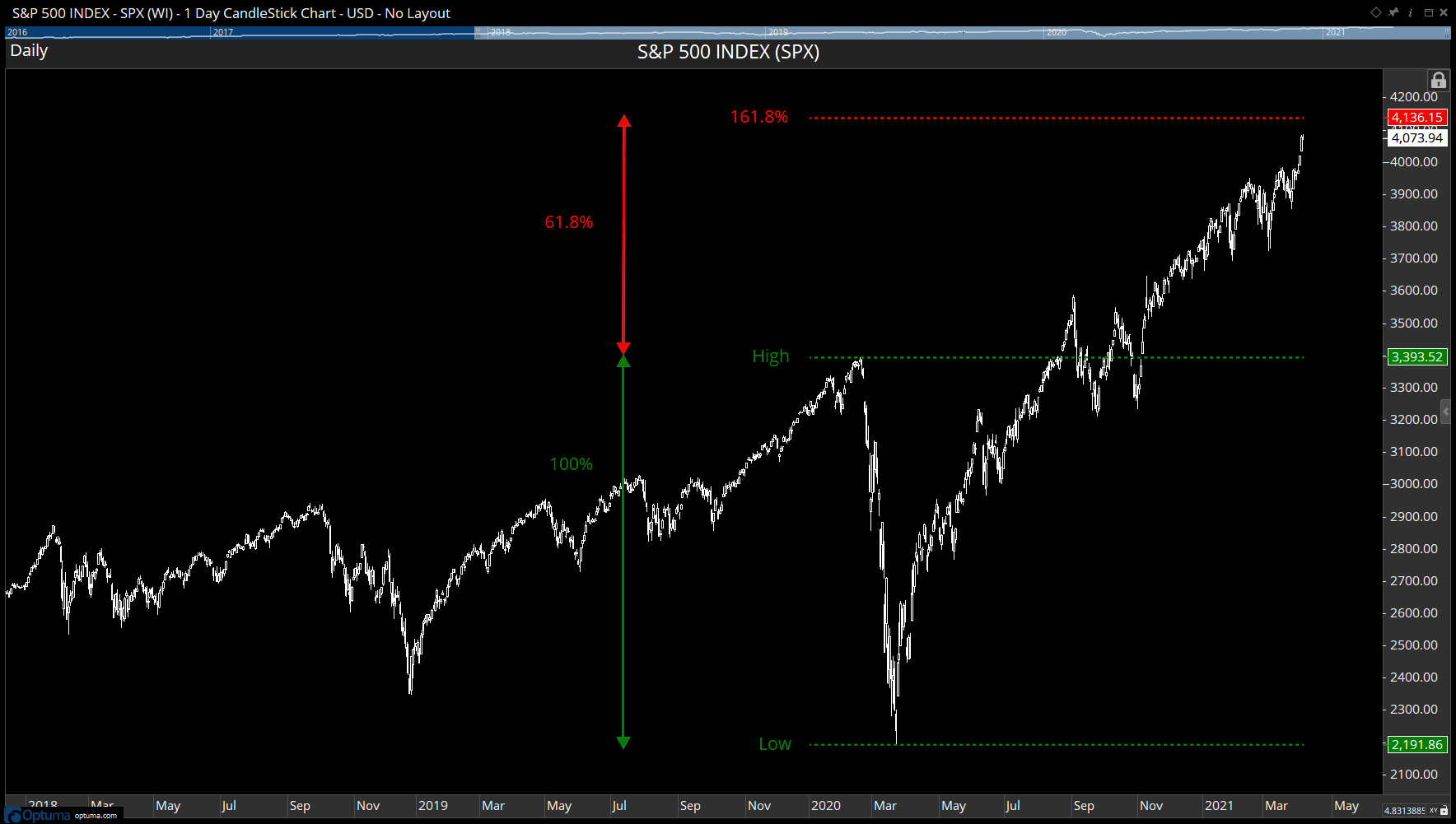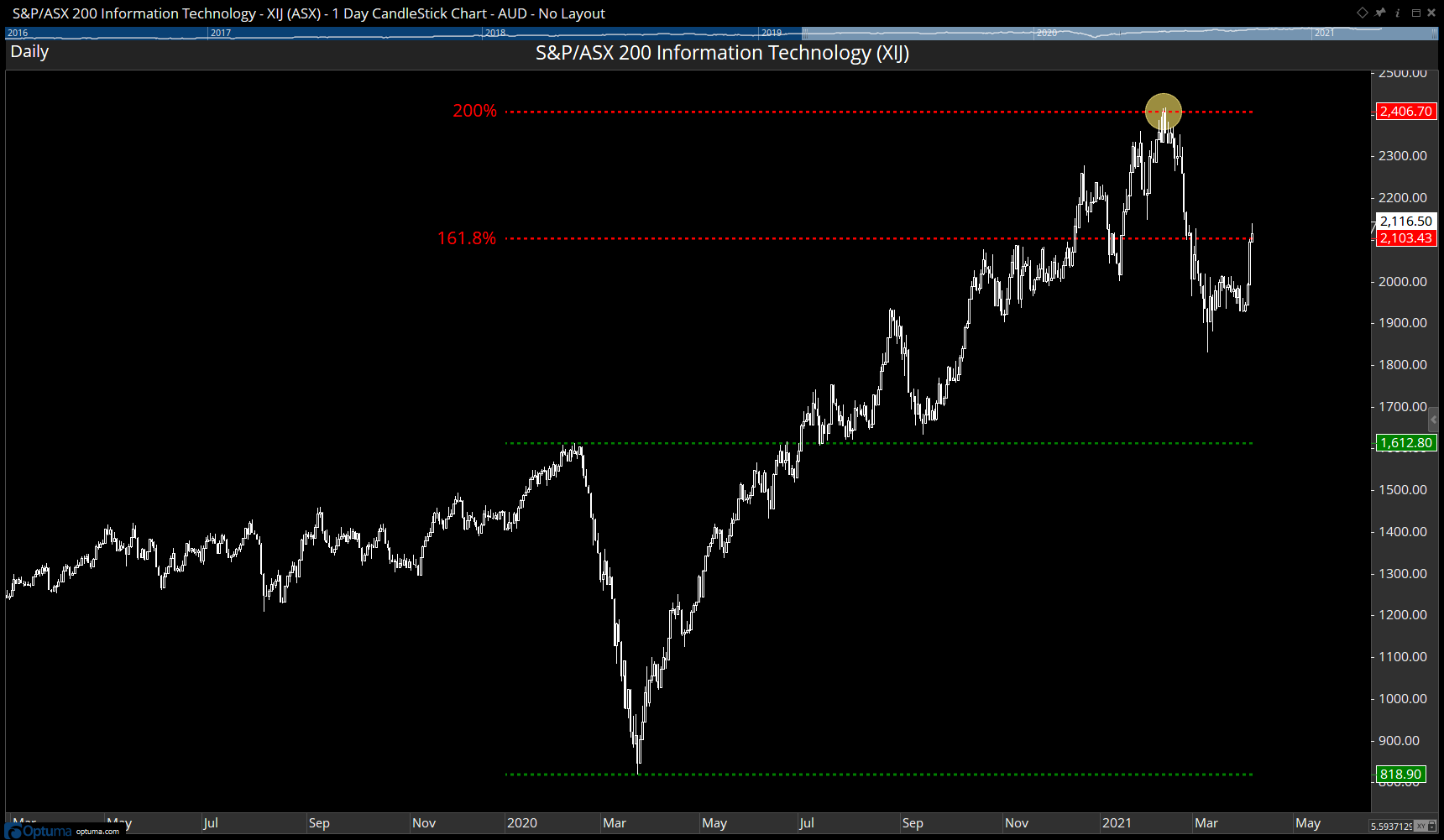Select Page

Optuma Forums Optuma Scripting Calculating Retracements Reply To: Calculating Retracements

#63369

This retracement formula can be used to plot the levels on a chart, and from that its possible to plot any other level, such as the 161.8% Fibonacci extension level, or 200% measured move of the original price drop.

The following will draw the high and low values, along with the 161.8%, 200%, and 261.8% levels of the 2020 COVID Crash:

To add extra levels duplicate from Line 26 down, change the formula in the next line, eg R1*3 for 300%, and replace Plot5 to Plot6.

Here’s an example of the S&P500 index approaching the 161.8% level at 4,136:Here’s the ASX200 IT sector (XIJ) showing the reversal at the 200% level (2,406) in February 2021, and is currently trading at the 161.8% level: